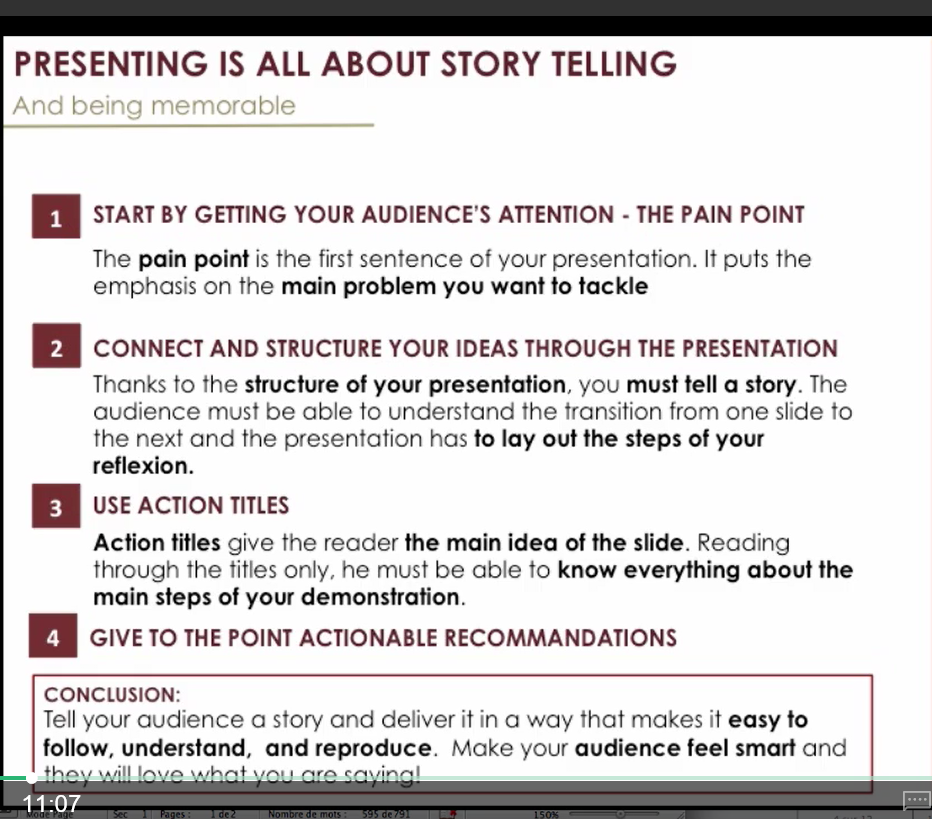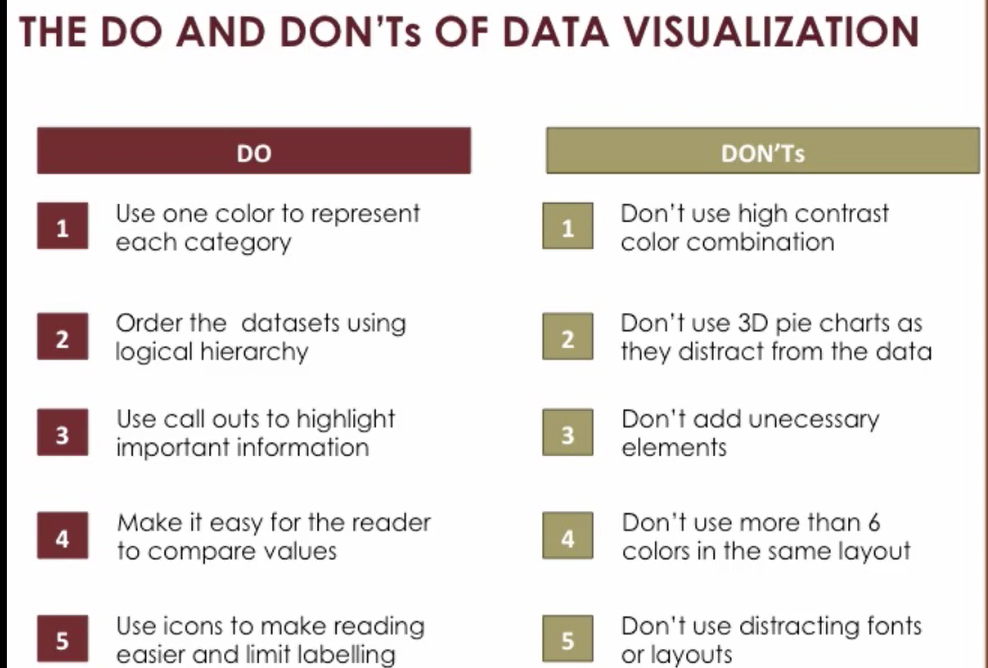# 【学习笔记】Foundations of Strategic Business Analytics

WEEK 1 Finding groups in data
• The objective of finding groups in data: find the right balance between similarities and differences.
• treat the silimar cases in a similar way to benifit from economies of scale.
• treat the different cases in a different way to improve actions' effectiveness.
• Basic cluster using as-hoc techniques
• SKU- stock keeping unit (using BCG matrix)
• x-axis: volatility of sales
• Horses: high sales and low variability, strong and reliable
• Bulls: high sales and high variability, strong but difficult to control --case to case
• Crickets: low sales and low variablity, small but can jump unexpectedly --make to order
• Identifying groups within data: the intuition behind clustering
• Hierarchical clustering technique
• hclust function (by R), a given number of groups, identify the optimal clusters.
• maximize the similarity within the clusters
• maximize the dissimilarity between clusters
• similarity is measured with the distance between observations. eg: Euclidean distance
• Remark
• select the number of groups as a parameter of hclust, rely on common sense and business criteria to decide, not the technical criteria such as dendrogram.
• include as many dimensions as we want
• Steps of cluster analysis
• step 1: normalize the variables
• First, subtract to each value from each variable the average from this variable (mean-centering). Compute the average value of each of variables per segment.
• Then, divide by the standard deviation. -> make the distance along variables comparable.
• step 2: apply hierarchical clustering
• how to decide the number of segment???
• step 3: interpret the result, how the segment are different
• ANOVA, analysis of variance
• Or just try to visualize the most noticeable difference with colors.
• aim at the best balance between cost and effectiveness.
• Recital
• SKU example: 将observations聚类，分组
• Hierarchical clustering method
• Step 1: normalize the data -- scale function
• Step 2: compute the distance between points -- dist function
• Step 3: perform the actual hierarchical cluster -- hclust function
• Step 4: assisn all of obversation to groups -- cutree function
• Step 5: visualization -- lattice package
testdata = scale (testdata)
d = dist (testdata, method = "euclidean")
hcward = hclust (d, method = "ward.D")
data$groups <- cutree (hcward, k=3) #data中新加一列groups，保存每个observation的组数 install.packages("lattice") library(lattice) xyplot(ADS ~ CV,main = "After Clustering", type="p",group=groups,data=data, # define the groups to be differentiated auto.key=list(title="Group", space = "left", cex=1.0, just = 0.95), # to produce the legend we use the auto.key= list() par.settings = list(superpose.line=list(pch = 0:18, cex=1)), # the par.settings argument allows us to pass a list of display settings col=c('blue','green','red')) # finally we choose the colour of our plotted points per group • HR example：given离职人的属性，找出人离职的原因 • Step 5：在SKU example Step 4的分组之后，in order to output the mean tables, using aggretate function to calculate mean aggdata = aggregate(.~ groups, data=data, FUN=mean) # The aggregate() function presents a summary of a statistic, broken down by one or more groups. Here we compute the mean of each variable for each group. • aggregate前，分组后的源数据ds •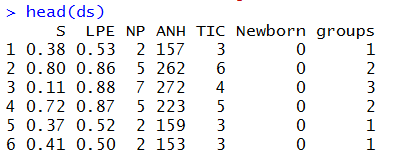• 按组进行每个变量的平均值计算后的数据 •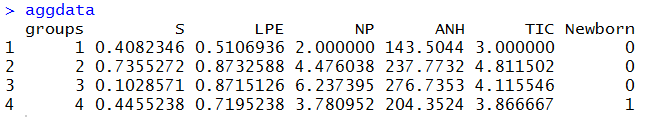• Step 6: compute the number of observation in each group (eg: using variable S) • Step 7: add the proportion variable to aggdata, by computing the ratio between the number of observation in each group. • Step 8: order the groups decreasely • Step 9: view data 生成表格 proptemp = aggregate (S~ groups, data=ds, FUN= length) aggdata$proportion = (proptemp$S)/sum(proptemp$S)
aggdata=aggdata[order(aggdata$proportion,decreasing=T),] View(aggdata) • 发现一整组数据中newborn为1，其他组为0，想找newborn是否与leave有关，新建数据，排除newborn属性，重复。 • 将分析后的数据存入csv文件供可视化使用 write.csv(aggdata, "HR_example_Numerical_Output.csv", row.names=FALSE) • Telecom example: customer segmentation, find the groups of clients that use services in simalar ways • 步骤与example2相同，最后画雷达图 palette(rainbow(12, s = 0.6, v = 0.75)) # Select the colors to use stars(aggdata[,2:(ncol(data))], len = 0.6, key.loc = c(11, 6),xlim=c(2,12),main = "Segments", draw.segments = TRUE,nrow = 2, cex = .75,labels=aggdata$groups)

WEEK 2 Factors leading to events
• Understand the causes and consequences
• rigorous statistical methods
• linear regression
• logistic regression
• correlation is not the same as causation
• what's the explanatory powerof the model?/ Is the model helpful in describling the situation?
• what are the significant factors?
• p-value: a measure of how weak the significant is. P值<0.05，显著，相关。p值越小，认为样本中变量的关联是总体变量关联的可靠指标。
• t-value: assess the strength of the significance of the driver. The larger the absolute value, the better.
• the sign of the estimates effect
• report the most significant revalant information to the manager in a visual way by just focusing on how important the factors are.
• ranking the factors and categorizing them with colors -> table
• plots
• understanding what distinguishes two categories
• observe both categories, for example, people who leave the company and people who stay (HR analytics example)
• compute the rate of people who leave the company
• perform a correlation with the cor command in R to see what are variables correlated the fact that an emplyee left the company
• logistic regression
• R: glm function, estimate the relationship between the event
• produce a similar output as a linear regression, except that we are interested in an outcome that is 0 or 1 and not continuous.
• compute the proportion of correctly classified observations for instance
• as the number of observations increase, the effects all tend to be significant, statistical significance usually becomes meaningless.
• the larger the absolute z-value is, the most important varibale is.
• what did we do to report the result of the regression
• Step 1-report accuracy: provide some numbers reporting clearly the accuracy the model
• error 1-false positive: the model estimated that someone should have left but she didn't
• erroe 2-false negative: the model failing to estimate correctly that someone has left
• Step 2-report a table focusing the effects that are significant or not
• in the red, report the "bad effects". Those impacting negatively what we want the outcome to be: for example we want to retain the employees.
• in the green, report the "good effects".
• loop of causality -> endogeneity
• Beyond the regression estimates: reporting the effects in a visual way
• use a plot instead a table to report the effects.
• model-free approach: we know what are the important effects, we can use method that does not rely on statistics
• Example: time/attrition
• use plot function in R to report the fact that the employees left or not.
• SUMMARY-Produce the results
• Step 1: build a model and make sure that the model is reliable
• Step 2: identify the most important factors and report the relationship with the outcome visually
• Step 3: use a   representation of the results. using visual clues which allows the observe complex effects instead of aggression.
• Recital
• 直方图--hist($a) 参数直接写变量，x轴变量区间，y轴frequency • Only numerical variables make sense to find the correlations between variables. • cor function: correlation only give information about the strength and the direction of the linear relationship between two variables. • glm function: is used to fit generalized linear models, specified by giving a symbolic description of the linear predictor and a description of the error distribution. • Warm-up • correlations didn't allow us to consider the joint effects of different rivals all together, and it didn't allow either to distinguish the most important effects from the non-signifincant ones. • need to estimate statistical models to be able to measure those relationships in a rigorous and quantitative way. • four steps to approaching such models • Step 1: assessing whether the resulting model is accurate • Step 2: if the resulting model is accurate, what are the significant factors? • Step 3: for those factors, can we rank by order of importance? • Step 4: MOST IMPORTANT. since they are part of a key message, we need to find a visual way to report the relationship between the driver and the outcome we are interested in. WEEK 3 • post hoc: 方差分析后的组间比较 • ergo propter hoc • focus on 3 approaches • how to predict which observations (customers, employees, etc.) are more likely to behave in a • when is a certain event likely to happen precisely? • how to model seasonal effects. • Using • HR example • use the predict on the output of the GLM function (used to conduct a logistic regression). • The difference between fitted function and predict function: • fitted是拟合值，模型是根据给定样本建立的，在给定样本上做预测是拟合，求出给定x的对应y值。 • predict是预测值，模型是根据给定样本建立的，在新样本上做预测是预测，求出新x的对应y值。 • then have for •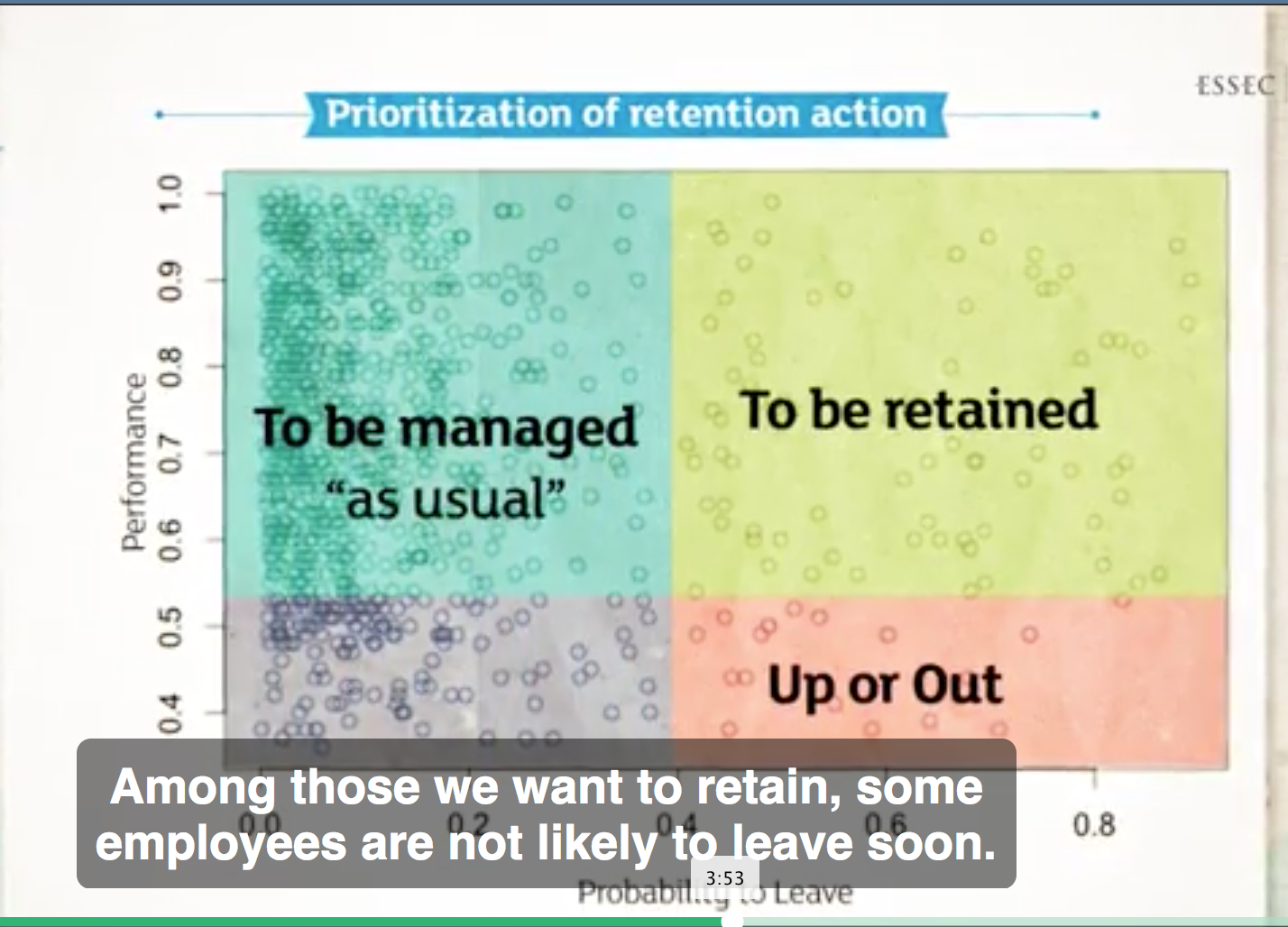• have a priority score on the employee, rank the priority score. The score is the combination of who is likely to leave and how much we want to retian them. •• Predicting when an event will happen with survival analysis • model how long before a customer churns （搅拌，买卖） • how long before a lender defaults（未履行，拖欠） • example in predictive maintenance: how long before a certain mechanical element breaks down • dataset: lifetime (in weeks), broken (0: still working, 1: broken), pressure, moisture, temperature, maintain_team, provider • if rely on the standard linea regression to find the causes leading to a failure, it's not correct or accrute. Because there are a lot of parts doesn't broken, and we don't know how long they will last. • p - value means the signaficant variables, the smaller(<0.05) the better. Estimate value (sine of the coefficient) means whether the effect is positive or negative for the excepted lifetime. Positive means •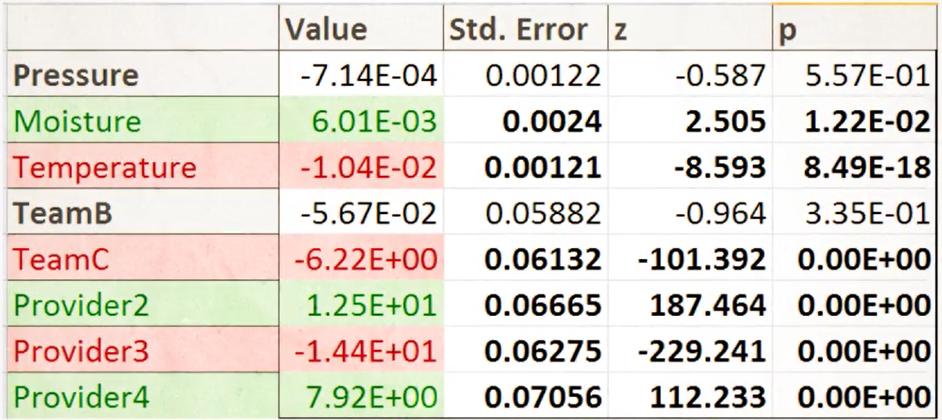• Introduction to time series and seasonality • Example: chocolate sale, peaks and bottom • estimate what the effect of month, using a simple regression where the sales woule be the dependent variable. • Many month have very strong effects on sales. The * means the variable had signicant effects on the outcome and *** means that the effect is strongly significant. • Estimate value (sine of the coefficient) shows that February, November and December have on average a positive effect on sales. While June to Sep. report a decrease of the sales. •• Visual way to report the results -- box plots to report the distribution of sales per month. 优点：compare the difference among all month. • Recital • Credit score example • build classification and regression models to make predictions (predict credit score). #estimate a linear regression model linreg = lm(Rating ~., data = olddata) #obtain predictions made by the model on newdata, 在newdata上做预测是预测，在建模的data上做预测是拟合。 predcreditscore = predict(linreg, newdata = datanew, type = "response") #检查拟合值和建模dataset的实际值的相关度 cor(lingre$fitted.values, dataold$Rating) #检查预测值和test set的实际值的相关度 cor(precreditscore, datanew$Rating)
• If we had more observations, we could give them a credit score and assess whether or not it makes sense to grant them a loan from a business point of view. -- 征信
• HR example
• want to see the relationship between a brunch of variables, and find out whether the employee left the company or not.
• build a logistic regression model, and use this model on the employees who are currently still with us to see the probility that they are leaving, in order to undertake actions aimed at retaining them.
• The difference of simple linear regression model and logistic regression model: 简单线性模型适用量化的解释变量预测量化的响应变量；逻辑回归模型是用解释变量预测一个类别型的响应变量（二值相应变量，0 or 1），即分类。
#logistic regression model预测分类，family是概率分布，分布族是binormal，默认连接函数是logit
logreg = glm(left ~., family = binormal(logit), data = dataold)
#assess that the model is performing well, then use it to make predictions for out-of-sample data
probaToLeave = predict(logreg, newdata = datanew, type = "response")
#structure the prediction output in a data frame
predattrition = data.frame(probaToLeave)
#add a column to the preadttrition data frame containting the performance (LPE: last project evaluation),生成了一个关于LPE和probaToLeave关系的表
predattrition$performance = datanew$LPE
• plot function画出来predattrition to find out the employees we want to retain.
•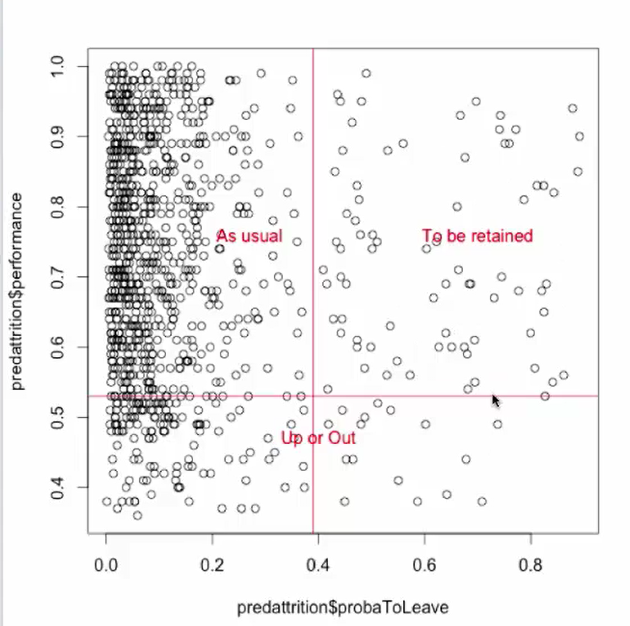• Another way to prioritizing employees:
#establish a priority score by multiplying performance and probability to leave for each employee
prebattrition$priority = prebattrition$performance * preattrition$probaToLeave #order the employeees by priority orderprebattrition = prebattrition[order(preattrition$priority, decreasing = TRUE),]
• Predictive maintenance example
• perform a survival analysis to estimate the remaining lifetime.
• Linear regression model: using all of variables except broken. But use the linear regression model in this case is inaccurate. Because most of the observations are not broken,
linregmodel = lm(lifetime ~.-broken, data = data)
• right-censored problem: when the event to happen hasn't necessarily happend ready.
#Step 1: set dependent variables
dependantvars = Surv(data$lifetime, data$broken)
#step 2: build a regression model
survreg = survreg(dependantvars ~ ...+...+..., dsit = "gaussian", data = data)
#step 3: assess the model on out of sample data,不能使用不保证准确的模型，或者不知道limits的模型。
#step 4: using the model to estimate the remaining lifetime of machines, which are not currently broken.返回lifetime的期望(p = .5)
Ebreak = predict(survreg, newdata = data, type = "quantile", p = .5)
#step 5: build the dataframe
forecast = data.frame(Ebreak)
forecast$lifetime = data$lifetime
forecast$remainingLT = forecast$Ebreak - data$lifetime #step 6: optimize the output forecast = forecast[order(forecast$remainingLT),]
actionpriority = forecast[forecast$broken = 0,] • Chocolate sales forecasting example • time series的sample要考虑好时间粒度 #step 1: plot the sales as a function of time to get a better intrition of data (shows seasonality), ylm argument is used to set a specific limit to the y axis wider than the default by multiply the max value. plot(data$time, data$sales, ylm = c(0, max(data$sales * 1.2)), type = "l")
#step 2: build a linear regression model.
regres = lm(sales ~ month, data = data)
#step 3: create plot to see the distribution of sales for each month. The month variable is a factor, so R will automatically represent the sales per month using box plot.
plot(data$month, data$sales, ylm = c(0, max(data$sales * 1.2))) #step 4: test the model on past data. Plot the actual sales and add the sales as provided by the model (in blue) in the same plot, then add a legend. plot(data$time, data$sales, ylm = c(0, max(data$sales * 1.2)), type = "l")
lines(data$time, regres$fitted.values, type = "l", col = "blue", lty = 2) --dash line
legend("topleft", c("Actual sales", "Sales by the model"), lty = c(1, 2), col = c("black", "blue"))

WEEK 4 Recommendation production and prioritization
• present the results in a visual, relevant and insightful way.
• present the summary statistics to diffrent audience
• how to present business analytics work to a business audience: find an angle and tell a story
• best presentations always share characteristics, stroy driven.
• shouldn't focus on what you want to say about your work: how technical it was, how difficult some of the analyses were
• focus on the key messages you want to convey to your audience
• a good business analytics story has a good beginning, starting the prensentation with a relevent, to the point, hookup. Directly identify the issue at hand in a qunantitative and visual way.
• the key issue of the presentation is very often discovered the other way around.
• Recommendations should focus on most efficient action to take. Make sure to prioritise actions accroding to their efficiency.
• First,
• Then, present the action on the longer run.
• Discard the less impactful or unrealistic recommendaions.
• Connect logically conclusions
• need to explain why you need
• why the methodology you've chosen is revelent
• One slide / One idea
• summarize as much information as possible, while keeping the right banalce of how clear they are.
• if see slides as the support oral presentation -> as few text as possbile, use image
• if see slides as the written document -> self-contained and presnets first and foremost information. (typical consultant approach)
•